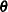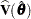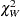### Testing Linear Hypotheses about the Regression Coefficients

Linear hypotheses forare expressed in matrix form aswhereis a matrix of coefficients for the linear hypotheses andis a vector of constants. The vector of regression coefficientsincludes slope parameters as well as intercept parameters. The Wald chi-square statistic for testingis computed aswhereis the estimated covariance matrix in the section Variance Estimation. Under,has an asymptotic chi-square distribution withdegrees of freedom, whereis the rank of.